# Simplified Common Emitter Hybrid Model Electrical Engineering (EE) Notes | EduRev

## Electrical Engineering (EE) : Simplified Common Emitter Hybrid Model Electrical Engineering (EE) Notes | EduRev

The document Simplified Common Emitter Hybrid Model Electrical Engineering (EE) Notes | EduRev is a part of the Electrical Engineering (EE) Course Electronic Devices.
All you need of Electrical Engineering (EE) at this link: Electrical Engineering (EE)

Simplified common emitter hybrid model:

In most practical cases it is appropriate to obtain approximate values of A V , A i etc rather than calculating exact values. How the circuit can be modified without greatly reducing the accuracy. Fig. 4 shows the CE amplifier equivalent circuit in terms of h-parameters Since 1 / hoe in parallel with RL is approximately equal to RL if 1 / hoe >> RL then hoe may be neglected. Under these conditions,

Ic = hfe IB .

hre Vc = hre Ic RL = hre hfe Ib RL .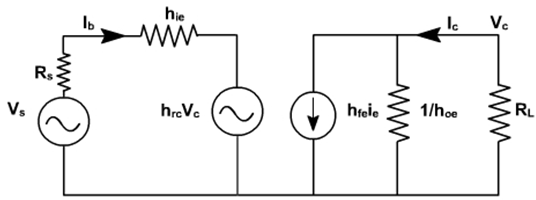Fig. 4

Since hfe.hre = 0.01(approximately), this voltage may be neglected in comparison with hie Ib drop across hie provided RL is not very large. If load resistance RL is small than hoe and hre can be neglected.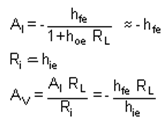Output impedance seems to be infinite. When Vs = 0, and an external voltage is applied at the output we find Ib = 0, I C = 0. True value depends upon RS and lies between 40 K and 80K.

On the same lines, the calculations for CC and CB can be done.

CE amplifier with an emitter resistor:

The voltage gain of a CE stage depends upon hfe. This transistor parameter depends upon temperature, ageing and the operating point. Moreover, hfe may vary widely from device to device, even for same type of transistor. To stabilize voltage gain AV of each stage, it should be independent of hfe. A simple and effective way is to connect an emitter resistor Re as shown in fig. 5. The resistor provides negative feedback and provide stabilization.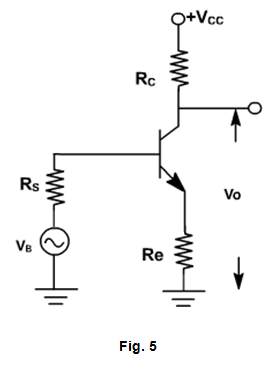An approximate analysis of the circuit can be made using the simplified model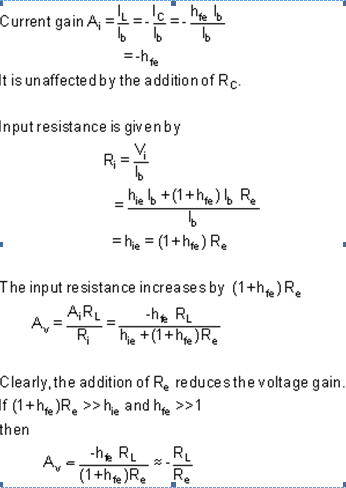Subject to above approximation A V is completely stable. The output resistance is infinite for the approximate model

Comparison of Transistor Amplifier Configuration

The characteristics of three configurations are summarized in Table. Here the quantities Ai, Av, Ri, Ro and AP are calculated for a typical transistor whose h-parameters are given in table .The values of Rand Rare taken as 3KΩ.

Table: Performance schedule of three transistor configurations.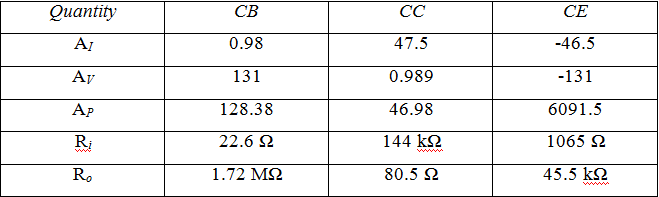The values of current gain, voltage gain, input impedance and output impedance calculated as a function of load and source impedances.

Characteristics of Common Base Amplifier

1. Current gain is less than unity and its magnitude decreases with the increase of load resistance RL,
2. Voltage gain AV is high for normal values of RL,
3.  The input resistance Ris the lowest of all the three configurations, and
4.  The output resistance Ris the highest of all the three configurations.

Applications: The CB amplifier is not commonly used for amplification purpose. It is used for

1. Matching a very low impedance source
2. As a non inverting amplifier to voltage gain exceeding unity.
3.  For driving a high impedance load.
4. As a constant current source.

Characteristics of Common Collector Amplifier

1. For low RL (< 10 kΩ), the current gain Ais high and almost equal to that of a CE amplifier.
2. The voltage gain AV  is less than unity.
3.  The input resistance is the highest of all the three configurations.
4. The output resistance is the lowest of all the three configurations.

Applications: The CC amplifier is widely used as a buffer stage between a high impedance source and a low impedance load.

Characteristics of Common Emitter Amplifier

1. The current gain Ais high for RL < 10 kΩ.
2. The voltage gain is high for normal values of load resistance RL.
3.  The input resistance Ri is medium.
4. The output resistance Ro is moderately high.

Applications: CE amplifier is widely used for amplification.

Offer running on EduRev: Apply code STAYHOME200 to get INR 200 off on our premium plan EduRev Infinity!

## Electronic Devices

53 videos|56 docs|97 tests

,

,

,

,

,

,

,

,

,

,

,

,

,

,

,

,

,

,

,

,

,

;